Students can go through AP Board 6th Class Maths Notes Chapter 9 2D-3D Shapes to understand and remember the concepts easily.

## AP State Board Syllabus 6th Class Maths Notes Chapter 9 2D-3D Shapes

→ Space: A plane is a set of all points which extends in all directions endlessly in three dimensions.

→ Plane: A plane is a smooth surface which extends in all directions in two dimensions.
Eg: The surfaces of a table, the surface of a blackboard are examples for part of a plane.

→ Polygon: A simple closed figure formed by line segments is called a polygon.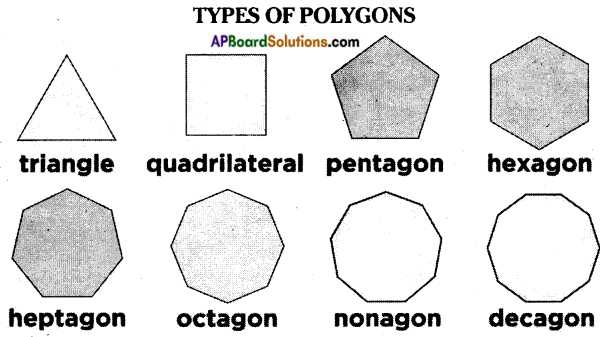→ Triangles:
A simple closed figure formed by three line segments is called a triangle.
The line segments $$\overline{\mathrm{AB}}$$, $$\overline{\mathrm{BC}}$$ & $$\overline{\mathrm{CA}}$$ are called the sides of the triangle.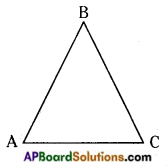A triangle contains three sides.
A triangle contains three interior angles ∠A, ∠B & ∠C and three vertices A, B & C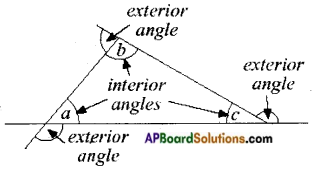→ A triangle divides the plane on which it lies into three sets of points.
1. Interior points on the triangle
2. Points on the triangle
3. Points in the exterior of the triangle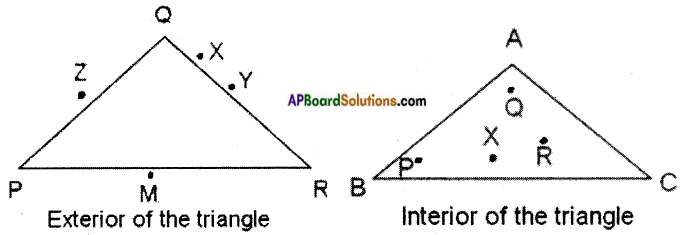A simple closed figure bounded by four line segments is called a quadrilateral.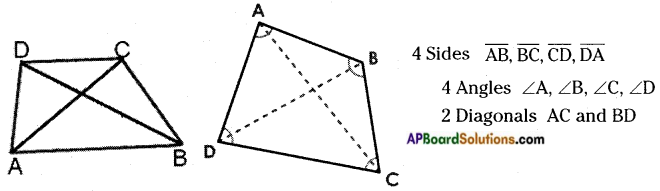→ Circle: If we draw a boundary along the edge of a round shaped object, then we get the following shape. The shape is called a circle. The length of the curved edge is called circumference. “A” is called the centre of the circle.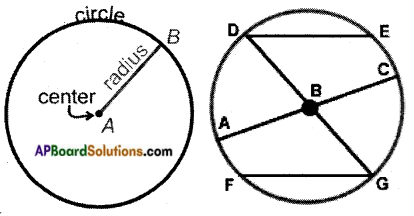→ Chord: A line segment joining any points ‘A’ & ‘B’ on the circumference of the circle is called a chord. DE and FG are chords of the circle.

→ Diameter: The longest chord passing through the centre of the circle is called a diameter. AC & DG are diameters.

→ Arc: A part of the circle is called an arc.

CIRCLE TERMINOLOGY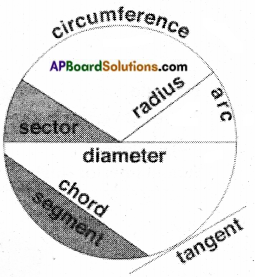→ Circumference: The distance around a circle.

→ Radius: The distance from the centre of a circle to the circumference. Half the diameter.→ Diameter: A straight line passing through the centre of a circle to touch both sides of the circumference. Twice

→ Chord: A straight line joining two points on the circumference of a circle. The diameter is a special kind of chord.

→ Arc: A section of the circumference.

→ Sector: A section of a circle, bounded by two radii and an arc.

→ Segment: A section of a circle, bounded by a chord and an arc.

→ Tangent: A straight line touching the circumference once at a given point.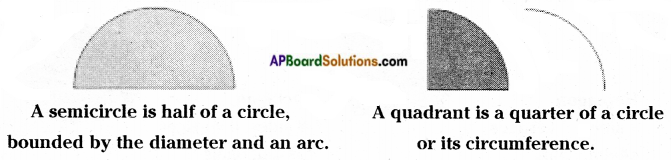→ A circle divides the plane on which it lies into three parts.

1. Interior points
2. Exterior points
3. Points on the circle

→ BASIC PARTS OF A CIRCLE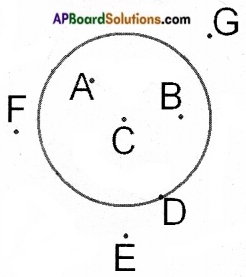• Interior of a Circle
points A, B, C
• ON the circle
point D
• Exterior of a Circle
points E, F, G→ The region in the interior of a circle enclosed by its boundary is called circular region.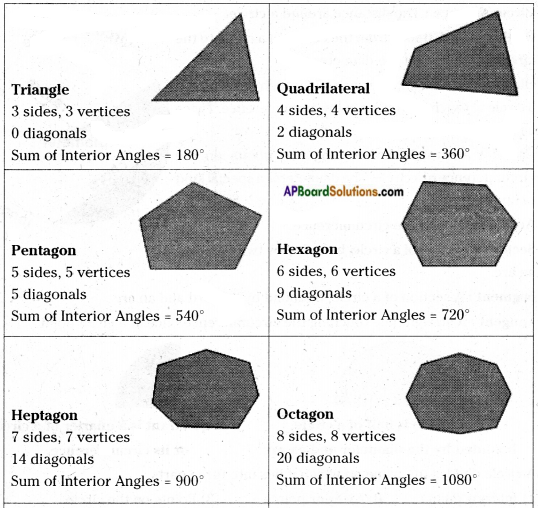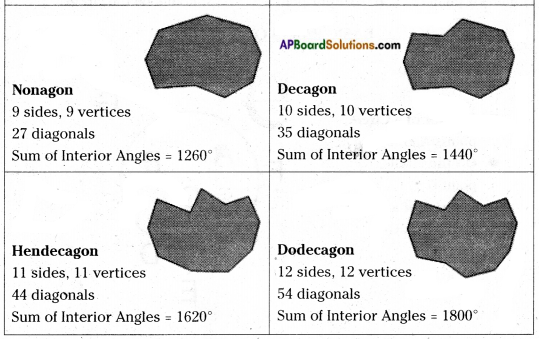→ Symmetry: Some figures appear beautiful because of their symmetry. Such shapes can be divided into two identical parts along a straight line which is called line of symmetry. A symmetrical figure may have more than one line of symmetry.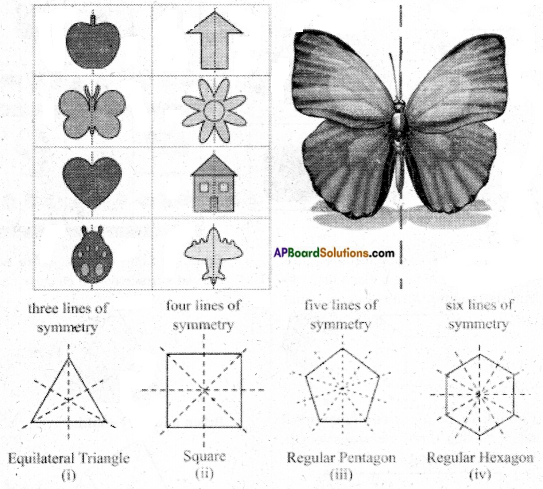→ English alphabet – lines of symmetry: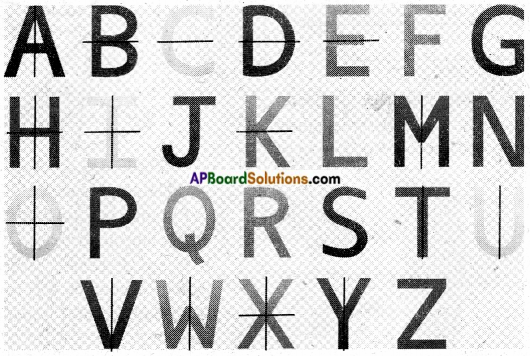3-D shapes:
→ NAMES OF 3D SHAPES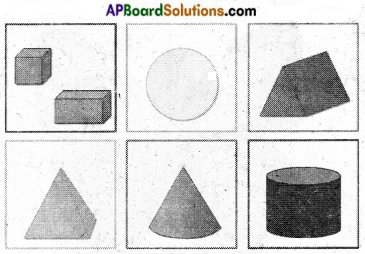→ 3D SHAPES IN REAL – LIFE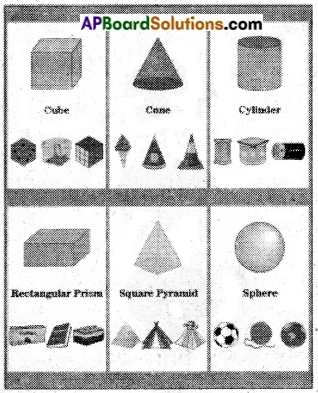→ Cube: A cube is a 3-dimensional figure. It has 6-identical faces. Each face of a cube is a square. All its sides are equal.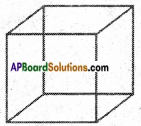→ Cuboid: A cuboid is three a dimensional figure having three measures length, breadth and height.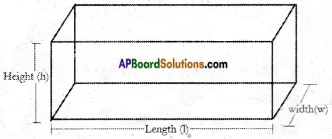→ Cylinder: A cylinder has circular faces at its both ends. It has two measures namely radius of the base and height of the cylinder.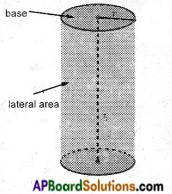→ Cone: A cone is a 3-d figure having curved surface with a circular base.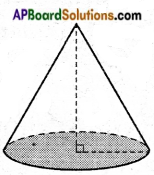→ Prism and a Pyramid: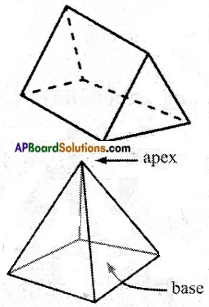A Prism is a 3-d shape with parallelograms as its lateral surfaces. A Pyramid is a 3-d figure with triangles as its lateral surfaces.
A Prism / Pyramid may have a triangle/ square/rectangle…as its base.
A Prism/Pyramid is named as per its base.→ Euler’s formula:
The number of faces (F), vertices (V) and edges (E) of a polyhedron are related by this formula: F + V = E + 2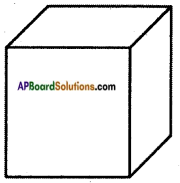Looking at the box to the right, calculate the number of edges:
Faces = 6
Vertices = 8
F + V = E + 2
6 + 8 = E + 2
14 = E + 2
E = 12